# Triangles, Circles, and Waves (oh my!)

### An Overview of TrigonometryCopyright (c) 1996 by Kenny Felder

Requires Fonts

This sheet is meant as a guide to help you review trigonometry. I assume you're already familiar with the basic concepts, and just need to have things cleared up or refreshed; if you've never seen trig before, this sheet will probably only be helpful in conjunction with a class.

### The Basic Idea

Start out by considering a right triangle: that is, a triangle with one 90o angle. The angle that we care about is one of the other ones, which we'll label with the Greek letter q. (Everyone always labels angles with Greek letters. Why? One loyal reader tells me this is done in honor of Pythagoras.) We're going to look at the sides: the big one, opposite the right angle, we call the hypotenuse. The other two don't have special names, but one is adjacent to q and one is opposite q, so those will do. In the triangle below, for instance, the side opposite q has length 2 (inches, or meters, or whatever). The side adjacent to q has length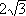. The hypotenuse, therefore, must have length 4 by the Pythagorean theorem.Now, we're going to start by defining the sine of q (written "sin q") as the length of the opposite side, divided by the length of the hypotenuse; so we'll say that sin 30o = (2 divided by 4) = 1/2. You might well ask why we would give a special name to such a thing. I'll be talking soon about how it can be practical and useful, but the key point to understand is that the sine of q depends only on q; it does not depend on the specific triangle. If you change the size of the triangle I drew up there, but leave the angles the same, the lengths of the sides will change, but the ratio sin q = opp/hyp will remain the same. So sin q gives us a way of representing the fact that all triangles that have the same angles, will have the same side ratios.

Look at the drawings below and see if you can convince yourself that sin q is the same for different triangles with the same q.Before I work an example in which the sine proves useful, let me mention the other two important trig functions. The cosine of q (write "cos q") is the adjacent side divided by the hypotenuse, and the tangent ("tan q") is the opposite side over the adjacent. You can memorize these by remembering the indian-sounding word SOHCAHTOA: it doesn't actually mean anything, except "Sine = Opp/Hyp, Cosine = Adj/Hyp, Tangent = Opp/Adj," but it is a hard word to forget if you say it out loud a few times. (Note: A couple of readers have pointed out alternative mnemonics: "Oh Heck, Another Hour Of Algebra" or "Oscar Had A Heap Of Apples." These are different tricks for memorizing the same thing: Opposite over Hypotenuse, Adjacent over Hypotenuse, and Opposite over Adjacent, for sin, cos, and tan, respectively).

Test yourself: looking at the triangles drawn above, what are the cosine and tangent of 30o? Check your answers on a calculator (but make sure it is in "degree" mode, instead of "radian" mode, or it won't work).

Knowing the meanings of the sine, cosine, and tangent, you can work most elementary trig problems if you have a calculator on hand. For instance, a typical problem might be: you want to measure the height of a lighthouse without climbing it. So, you stand thirty feet away from the lighthouse, and you measure an angle of elevation of 60o to the top. How tall is the lighthouse?

To start any such problem, draw a picture. Make sure you label all the relevant angles and sides.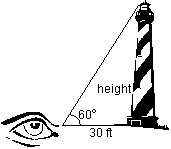Now, look at which sides are important. In this case, the side you know is adjacent to the known 60o angle; and the side you want to know is opposite the angle. This tells you which trig function you want: the tangent, since it relates the opposite and adjacent sides and ignores the hypotenuse. From here on, it's easy. Use a calculator or trig table to tell you that tan 60o = 1.73. Then write tan q = opp/adj and you can write the equation 1.73 = ht/30'. Solving, ht = 51.9 ft. Without leaving the ground, you have measured the height of a lighthouse very exactly: simply by knowing that the ratio tan q = opp/adj is the same for a gigantic lighthouse-person-cement triangle as for the little computer-drawn triangle on the page here! If you understand what we've done so far, you've got the basics; from here on in, it's just refinements.

### Trig Identities

Something most courses spend a lot of time on are the so-called "trig identities": that is, formulas that relate the various trig functions to each other. For instance, looking at the definitions sin q=opp/hyp, and cos q=adj/hyp, what is the ratio sin q/cos q? A little algebra will show you that this ratio equals opp/adj, which is just we've already defined as tan q! (Make sure you check the algebra here yourself; don't take my word for it.) So we have a trig identity: tan q = sin q/cos q. What makes this a trig identity is that it holds for any angle q; you might want to check this for yourself on a calculator for a few different angles.

The other always-useful trig identity is that if you take sin q and square it; and then you take cos q and square it; and you add these two things; you always get one. You might think this would be written like this:

`(sin q)2+(cos q)2=1`

But in fact, it is generally written like this instead:

`sin2q+cos2q=1`

So sin2q is just the way that people tend to write "sin q, squared," for some reason.

Anyway, I would encourage you to check this one out on a calculator too. Pick a number like 37, and type the following into your calculator.

`(sin(37))2+(cos(37))2`

and see if you always get 1. Then, for real fun, figure out why this particular relationship is true for any angle; this is pretty easy, using the definition of the sin and cos, and the pythagorean theorem. (I know, I know, you don't like doing proofs; but try this one anyway, honest.)

There are a million other trig identities, which enable you to rewrite sin(2q) or whatever; on the whole, you memorize these for the test and then forget all about them. Lord knows, that's what I do.

### The Unit Circle

The triangle picture of trig is very useful, but once we have it, we can discuss the trigonometric functions in a number of other useful ways. The two most popular are the unit circle, and the sine and cosine graphs. Each picture can be used to tell you different things about the trigonometric functions, but it's important to remember that all three pictures are describing the same sine, cosine, and tangent. So to start with, let's look at the unit circle.This drawing shows the unit circle; that is, a circle around the origin, with radius one. It also shows a line going from the origin, to some point on the circle. The angle from this line, to the x-axis, is labeled q. And there is a point, where the line crosses the circle, which I have labeled (x,y).

I've also drawn one other line, which goes from (x,y) straight down to the x-axis. I drew this vertical line because it gave me a little triangle. Now, based on our earlier triangle definitions, what is cos q? Well, cos q is the adjacent side over the hypotenuse, so look at the triangle in the drawing. The adjacent side is the x-coordinate of our point (x,y), which is just x. The hypotenuse is the radius of the circle, which is one. So the cosine is x/1, or x. You can just as easily prove to yourself that sin q is y. So, using the triangle definitions we discussed earlier, we have come to the circle definition of the trig functions:

Draw a line from the origin, at an angle q, and look at the point where that line intersects the unit circle. cos q is the x-coordinate of that point, and sin q is the y-coordinate.

This is a new definition of our trig functions; you can do everything based on this circle-definition instead of the triangle-definition, if you want to. But it should be clear from the above discussion that they are really the same thing.

What may not be clear is why you would want to have two different ways of defining the same thing, even if they do wind up being the same! The triangles were fine, why do we need circles? I think the biggest reason is that in our earlier definition, q was one angle of a right triangle, and such angles are always bigger than 0, and smaller than 90o. But on the circle, q can be anything: it can be much bigger than 90o, or it can even be negative!

For instance, what's sin 180o? Well, if you look at the 180o angle on the circle, it intersects at the point (-1,0). So cos 180o = -1 and sin 180o = 0.

When you get used to this, it can be a great time saver and boost your intuitive feel for the trig functions enormously. As a small test for yourself, what are the sine and cosine of 90o? (Check your answers on a calculator!) With a little practice you can do this one and a few other easy angles in your head, just by picturing the unit circle.

As another example of the usefulness of the unit circle, consider the sine and cosine of (-q). Well, on the unit circle below I've drawn in an angle q and its opposite (-q). You can see that the x-coordinates (the cosines) are the same and the y-coordinates (the sines) are opposite, so we get the trig identities:

cos (-q) = cos q and sin (-q) = -sin q.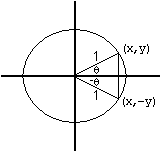Again, you might want to check these identities yourself using a calculator and a few simple angles. For instance, find the sine and cosine of 10o, then of -10o. Are the two cosines the same? Are the two sines opposite?

One more example of the usefulness of the unit circle: textbooks often give you an angle, and ask you if the sine and cosine are negative or positive. They encourage you to memorize rules about what is negative and positive in different quadrants, but there is really no need to memorize anything: you just need to picture the unit circle. For instance, suppose the angle is 110o. Well, you can see on the unit circle that this puts us in the upper-left-hand quadrant; so x is negative and y is positive; so cos 110o is negative, and sin 110o is positive. You can't figure out the exact values without checking your calculator; but if your calculator gives you a negative sine, you can bet you punched something in wrong!

Also, remember that tan q = sin q/cosq. So tan 110o will also be negative, since a positive number divided by a negative number will always give you a negative.

As with most things on the unit circle, this whole train of thought takes more time to write out than it takes to actually do it. Take a little time to work with the unit circle, until you can picture it in your head, and it will be a very great help to your understanding of the trig functions.

### Graphing the Sine and Cosine

Before you can fully understand the behavior of any sort of function, you have to graph it. In the case of the trig functions, the graph is pretty easy to draw if you look at the unit circle for a while. The strategy is simple: start out at q=0o, and follow the unit circle around, watching what the y-coordinate does (for the sine, that is; watch the x-coordinate for the cosine). I'm about to do the sine: follow me, around the unit circle, then try to do the cosine for yourself.Sin 0o is zero, as you hopefully figured out for yourself by looking at the unit circle last page. Sin 90o is 1. In between, as q moves from zero to ninety, the y-coordinate on the unit circle is constantly increasing. So the graph starts out like: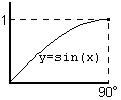Continuing to follow the unit circle around, as q increases to 180o the y-coordinate drops to zero: we're now at the point (-1,0) on the unit circle. As q keeps going from there, the y-coordinate doesn't turn around and go back up to one; it keeps going down, reaching -1 when q gets to 270o. Then the y-coordinate turns around, and reaches zero again when q is 360o; we are now, of course, at exactly the same place on the unit circle that we were at when q was 0o. So the full sine graph looks like: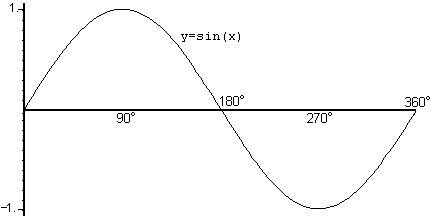You can keep on going from there around the unit circle, retracing the path around and around; so the graph repeats itself indefinitely.If you followed me carefully around the unit circle, all of that should have made sense. If you're like most people, you kind of fuzzed out half way; so do yourself a favor, and do the cosine graph for yourself. Start at 0o, where the x-coordinate is 1, and walk yourself all the way around the unit circle, following what the x-coordinate does from 0 to 90o, then to 180o, then to 270o, and finally back around to 360o, which is the same place on the circle as zero. See what the graph looks like for one full cycle, and convince yourself that it will now repeat itself indefinitely.

When we went from the triangle to the circle, I said that there were certain things that would be easier to understand with this new representation. Similarly, the graph gives us ways of understanding some aspects of the trig functions more directly than either the triangle or the circle. For instance, look at your sin and cos graphs side by side. Note that they look exactly the same, except that they are offset by 90o. This gives us yet another trig identity: sin q=cos(q-90o). Once again, you don't have to memorize this identity: you can easily recreate it, just by knowing what the graph looks like.

### We're Done!

What we've just covered is the three basic ways of looking at trigonometry: with triangles, circles, and the graph. If I've been successful, you now have a feeling that...
• The triangle representation of trig makes sense. That is, it makes sense to define these things called sine, cosine, and tangent, because no matter what triangle you draw with a 37o angle, the sine of 37 will always be the same.
• The circle representation of trig makes sense. That is, you can see that it really is another way of representing the exact same functions that we discussed on the triangle; and, you can see that there are certain kinds of things that are easier to understand on the circle than on the triangle.
• The sine and cosine graphs make sense. That is, you can see that these are yet another way of representing the exact same functions that we discussed on the triangle and the circle; and once again, you can see that there are certain kinds of things that are easiest to understand on the graph.
The next step is to start working problems. Some of them will require using trig identities that you just have to look up, and that's fine. But the basic ideas should all make sense.

### A Bit More Background Added By Suggestion

Mike Housky was kind enough to write to me and suggest the addition of some background material. The first paragraph I quote verbatim from him, since it is a very cool bit of trivia that I did not know, and I don't have anything useful to add to it.
"One thing that might help the opening is a discussion of the word 'trigonometry'. 'Trigon' comes from Greek roots meaning 'three sides' and is another name for a triangle. '-Metry' is a suffix meaning 'measurement' and the full term roughly translates to 'triangle measurement'. This could add some motivation—or perhaps just some added color—to the discussion of the triangle approach."
In addition, Mike goes on to suggest that "In the discussion of the unit circle approach (my favorite, by the way), some mention of radians seems appropriate." I think this is a great idea, so here I go! As you presumably know, angles are usually measured by a system where a right angle is 90o; so a straight line is 180o and the full circle is 360o. As far as I know, this is completely arbitrary; there is no good reason to call a right angle "90" of anything.

Radians provide a unit of measurement that is not completely arbitrary. When you measure in radians, a full circle is 2p, so a right angle is p/2. Now, this may seem like a big step in the wrong direction, but consider this: the circumference of a circle is 2p times the radius. A-ha!

The point is, on our unit circle, consider some angle q.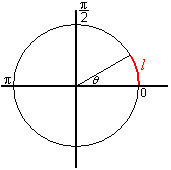In the drawing, I have labeled an angle q, and also the arc l along the circle from 0 to q. I think you'll agree that if q gets bigger, l also gets bigger. If q is 0, l is 0. What may not be quite so obvious, but I hope it makes sense, is that they are perfectly proportional: if q doubles, l doubles. If we measure q in radians, then the constant of proportionality is 1: in other words, the angle measurement (in radians) is the arc length. Because if we go around the whole circle, they both go to 2p.

So radians are just another way of measuring angles, just like degrees are. But with radians, you get the added bonus that the angle is the arc length—or, when it isn't a unit circle, the arc length divided by the radius of the circle. Pretty cool, huh?

### Want to Read Even More?

Kenneth Boyd has a much more detailed Trigonometry Crash Review that you can check out.Gary and Kenny Felder's Math and Physics Help Home Page
`www.felderbooks.com/papers`Send comments or questions to the author